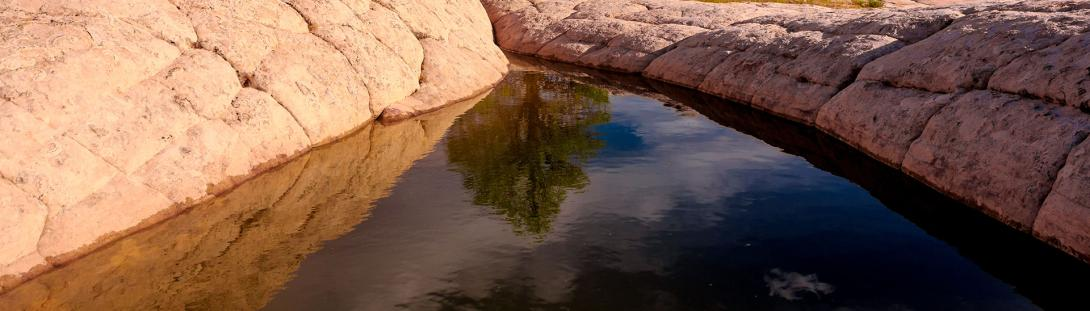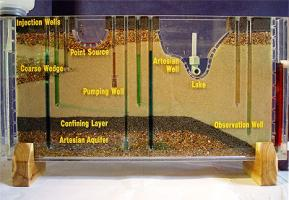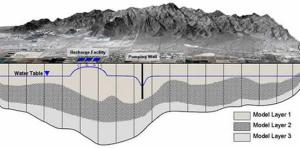## Modeling Basics

### What is a Model?Models serve as a conceptual representation of a physical reality. A model's representation can be physical, mathematical, or logical in form. Models can be found in all fields including financial, climatological, environmental, and engineering.

Models are developed from known conditions, typically in the form of measured data. Each model represents a conceptual representation of the processes being examined. Models can be used to simulate future conditions.

### What Types of Models are Used for Groundwater Evaluation?

The two types of models typically used to evaluate groundwater conditions in Arizona are analytical models (Winflow, Thwells) and numeric models (USGS MODFLOW).

Analytical models present a simplistic evaluation of an aquifer (single geologic unit, simplified aquifer parameters). Typically, analytical groundwater models utilize the "Theis" non-equilibrium radial flow equation. The Theis equation is a mathematical equation that treats the aquifer as a uniform porous media and can solve for future drawdown at a well, based on assumed aquifer parameters, boundary conditions, and projected pumping rates.

Numeric models have the ability to capture more of the aquifer's complexity. Numeric models solve groundwater flow equations by dividing an aquifer system into individual model cells that have characteristic aquifer parameters and pumpage assigned to each cell. The ADWR Groundwater Modeling Section uses the USGS 3D numeric groundwater flow model code to evaluate regional aquifer behavior in the ADWR-modeled areas of Arizona. (Not all aquifers within Arizona are modeled by ADWR; see  the map of ADWR models in the "Developed Groundwater Models" section for further details.)

### How are the ADWR Regional Models Used?

ADWR uses regional groundwater flow models to evaluate current conditions and simulate possible future scenarios. Each model cell within the numeric MODFLOW model represents conditions unique to part of the model. Components of the modeled area - including pumpage, recharge, evapotranspiration, streamflow, and flow - across model boundaries are simulated within each regional model developed by ADWR.